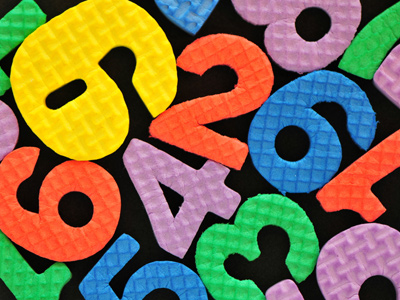You'll find a mixed bag of numbers to multiply in this times tables quiz.

# Times Tables (Mixed)

This Math quiz is called 'Times Tables (Mixed)' and it has been written by teachers to help you if you are studying the subject at elementary school. Playing educational quizzes is an enjoyable way to learn if you are in the 3rd, 4th or 5th grade - aged 8 to 11.

It costs only \$12.50 per month to play this quiz and over 3,500 others that help you with your school work. You can subscribe on the page at Join Us

This times tables quiz will ask you to multiply different numbers. This will help you to practice all the times tables you have been learning in your classes. Keep playing the times tables quizzes because learning the times tables by heart will make it easier to multiply different numbers together. This comes in very handy when dealing with figures in everyday life!

See how well you know your times tables by playing this quiz.

1.
12 x 9 =
92
99
108
120
To multiply any number by 9 just times it by 10 and then take it away, so 12 x 10 = 120 and 120 - 12 = 108
2.
8 x 11 =
80
84
88
92
To multiply a low number by 11 just use the same number twice, so 2 x 11 = 22, 4 x 11 = 44 and 8 x 11 = 88
3.
7 x 7 =
49
51
54
57
4.
9 x 7 =
61
63
65
68
5.
9 x 6 =
45
51
53
54
If you know your 9 times table or your 6 times table then you'll have gotten the answer straight away
6.
6 x 8 =
42
44
48
49
7.
8 x 8 =
54
57
64
67
A number times itself is called a square, so 8 squared is 64
8.
4 x 9 =
32
33
36
38
5 x 9 = 45 and 45 - 9 = 36
9.
5 x 8 =
40
42
45
46
To multiply a number by 5 first times it by 10 and then half it, so 8 x 10 = 80 and 80 ÷ 2 = 40
10.
6 x 7 =
38
42
46
48
Author:  Amanda Swift